# Estimating the Definite Integral Using Riemann Sums

Knoji reviews products and up-and-coming brands we think you'll love. In certain cases, we may receive a commission from brands mentioned in our guides. Learn more.

This article is written for two distinctive audiences: The first that wants to know the theory behind the answer versus the second group that is simply interested in the mechanics needed to get the answer. If mathematics is not your favorite subject, skip the discussion and go to the sample problem. The sample problem will show you how to estimate answers for curtain calculus problems without knowing how to solve a calculus problem!

The Theory

The discussion below will be a development of a technique to estimate the definite integral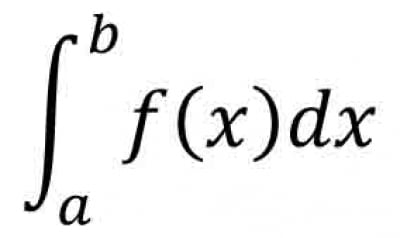when f(x), is such that f(x)>0 for all values of x, f(x) is continuous, and the function is increasing in the interval [a,b].

Note: The discussion will be presented in an intuitive and logical method versus a rigorous mathematical proof. Furthermore, the function will be arbitrarily placed in the first quadrant in the Cartesian coordinate system for ease in graphing.

Preliminary discussion: This section is included to take advantage of the simplicity that the initial conditions provide and to use that simplicity to estimate the definite integral.

The function is given as being both continuous (no discontinuities), greater than zero, and that the function is increasing. The notion that a function is increasing means that if you are given two arbitrary points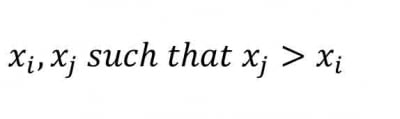The resulting values of the function will be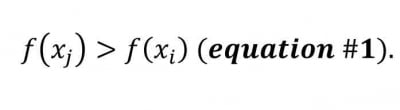This idea is important because the slope of the function will be positive and increasing. See Fig #1.Since f(x)>0, for all values of x from a to b, then the area under the function will be positive. That being said and noting that the integral defines the area of a function we have that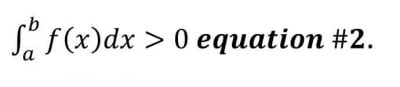Given what we know about the function we can create an arbitrary graph as presented in Fig #1.

To solve the problem we need to determine the area under the function. We want to find the easiest method by using a simple geometrical approximation. Let’s start by looking at Fig #1 and noticing we have two sets of graphs for the same function. In the first set we have rectangular boxes below the graph and in the second set we have rectangular boxes above the graph. We can create these rectangles by taking the two endpoints and dividing by the number of n rectangles we want to create. Thus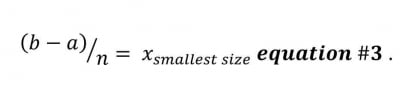Thus, the sum of all x' s = (b-a). From Euclidean Geometry we know that the area of a rectangle is defined as the length multiplied by the width. The width, in our figure, is the distance traveled from one value of x to the next value of x. Then the change in two subsequent values of x is defined as: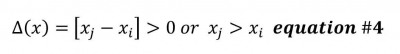Equation #4 is greater than zero because geometrically we are moving from the left side of the rectangle to the right side of the rectangle to calculate the area. The indices i and j simply represents any two adjacent values of x; for example the first value of x and the second value of x. We can calculate the value of f(x) for each value of x for both sets of rectangles. Thus the area becomes:The result is: One set of rectangles are those whose values are calculated with the smallest value of the function per a given interval (represented by the left graph of figure #1) and the second set are rectangles whose area is determined by the largest value of the function per a given interval (represented by the right graph of figure #1). Note: the left graph are all the rectangles (that touch the graph on the left) but whose sum are smaller than the area of the function while the graph to the right are all the rectangles (that touch the graph on the right) but whose sum are greater than the function.

Thus the total area can be found by adding all the rectangles in each category (those smaller than the function versus those larger than the function). We have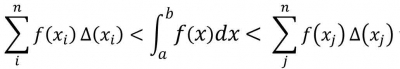(where n is the number of intervals we wanted) equation #7

As n gets larger and approaches infinity the difference becomes zero resulting in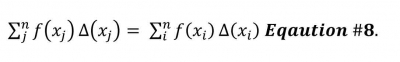Simply put: The area for all the left side rectangles equate the area for all the right side rectangles as you make the width of each rectangle smaller and smaller.

For simplicity let us define lower sum as L (called the lower Riemann limit) and the upper sum as U (called the upper Riemann limit). Thus we have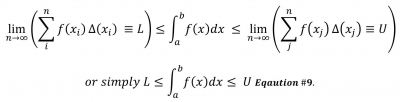This discussion is in essence what is called the Riemann Sum.

The Sample Problem

Let us look at a simple example to illustrate how to use Riemann Sums. Let us say you wanted to know the approximate area for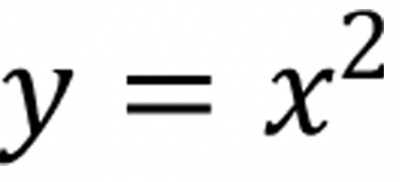starting from x = 0 to x = 5.

We can start simple and take n = 5. (Picking n to be the largest value of x in this case 5). We get the following graph. See Fig #2.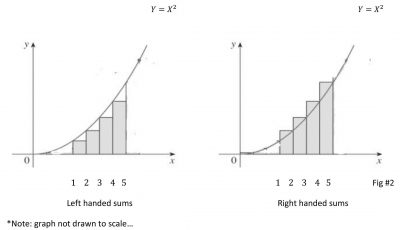First add the sum of all the rectangles to the left and then add the sum of all the rectangles to the right. The true answer is between these two sums.

The area for the left side is: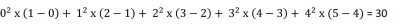The area for the right side is: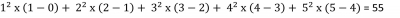Trick: For the left side start with the smallest value (in this case zero) and go to the second highest value (in this case 4). On the right side start with the next highest value from the bottom (in this case 1) and go to the highest value (in this case 5).